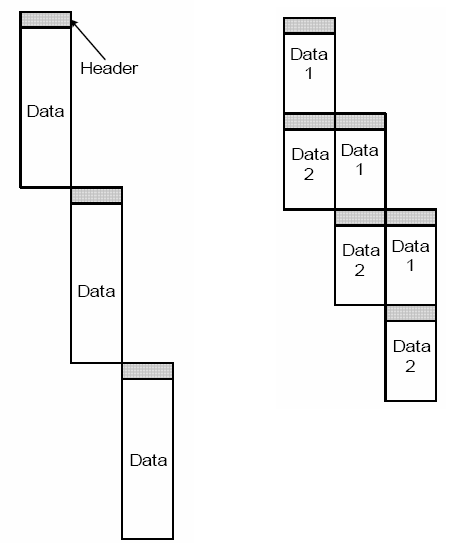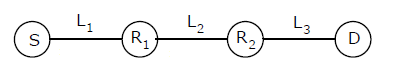GeeksforGeeks App
Open AppBrowser
Continue

# Computer Networks | Set 6

Following questions have been asked in GATE CS 2005 exam.

1) An organization has a class B network and wishes to form subnets for 64 departments. The subnet mask would be:
(a) 255.255.0.0
(b) 255.255.64.0
(c) 255.255.128.0
(d) 255.255.252.0

The size of network ID is 16 bit in class B networks. So bits after 16th bit must be used to create 64 departments. Total 6 bits are needed to identify 64 different departments. Therefore, subnet mask will be 255.255.252.0.

2) In a packet switching network, packets are routed from source to destination along a single path having two intermediate nodes. If the message size is 24 bytes and each packet contains a header of 3 bytes, then the optimum packet size is:
(a) 4
(b) 6
(c) 7
(d) 9

Dividing a message into packets may decrease the transmission time due to parallelism as shown in the following figure.But after a certain limit reducing the packet size may increase the transmission time also.

Following figure shows the situation given in question.Let transmission time to transfer 1 byte for all nodes be t. The first packet will take time = (packet size)*3*t. After the first packet reaches the destination, remaining packets will take time equal to (packet size)*t due to parallelism.

```If we use 4 bytes as packet size, there will be 24 packets
Total Transmission time = Time taken by first packet +
Time taken by remaining packets
= 3*4*t + 23*4*t = 104t

If we use 6 bytes as packet size, there will be 8 packets
Total Transmission time = 3*6*t + 7*6*t = 60t

If we use 7 bytes as packet size, there will be 6 packets
Total Transmission time = 3*7*t + 5*7*t = 56t

If we use 9 bytes as packet size, there will be 4 packets
Total Transmission time = 3*9*t + 3*9*t = 54t
```

3) Suppose the round trip propagation delay for a 10 Mbps Ethernet having 48-bit jamming signal is 46.4 ms. The minimum frame size is:
(a) 94
(b) 416
(c) 464
(d) 512